Question

# -Suppose the birth weights of full-term babies are normally distributed with mean 3700 grams and standard...

-Suppose the birth weights of full-term babies are normally distributed with mean 3700 grams and standard deviation of 490 grams.

a. Draw a normal curve with the parameters labeled and shade the region that represents the proportion of full-term babies who weigh more than 4680 grams.

b. Find the proportion of full-term babies who weigh more than 4680 grams.

-Find each of the following. Include a diagram for each:

a. Find the z-score such that the area under the standard normal curve to the left is 0.32

b. Find the z-scores that separate the middle 48% of the distribution from the area in the tails of the standard normal distribution.

c. Find ?0.38

Assume a random variable X is normally distributed with a mean ? = 50 and a standard deviation ? = 7. Compute the following probabilities. Be sure to draw the normal curve representing each situation.

a. P(X>34)

b. P(35

c. Find the 25th percentile.

Please show all work for the following problems. If a calculator is used, including the calculator function used as well as the values entered. Example: normalcdf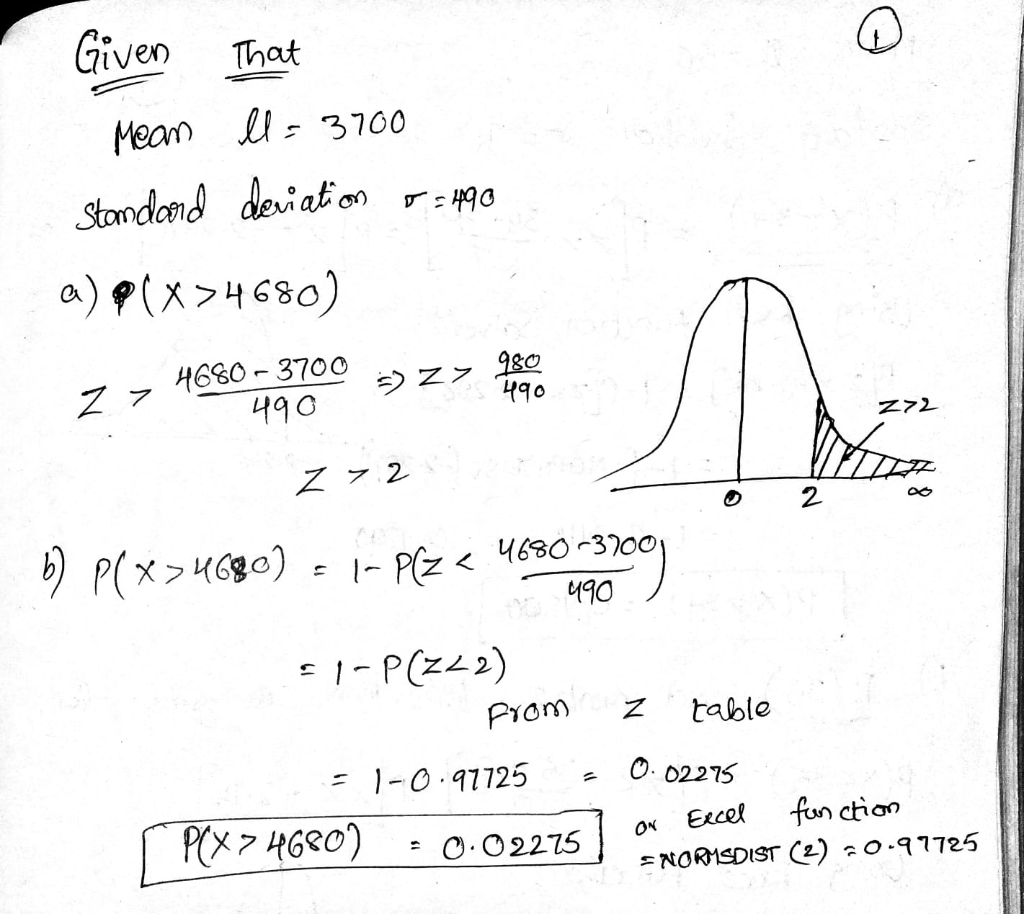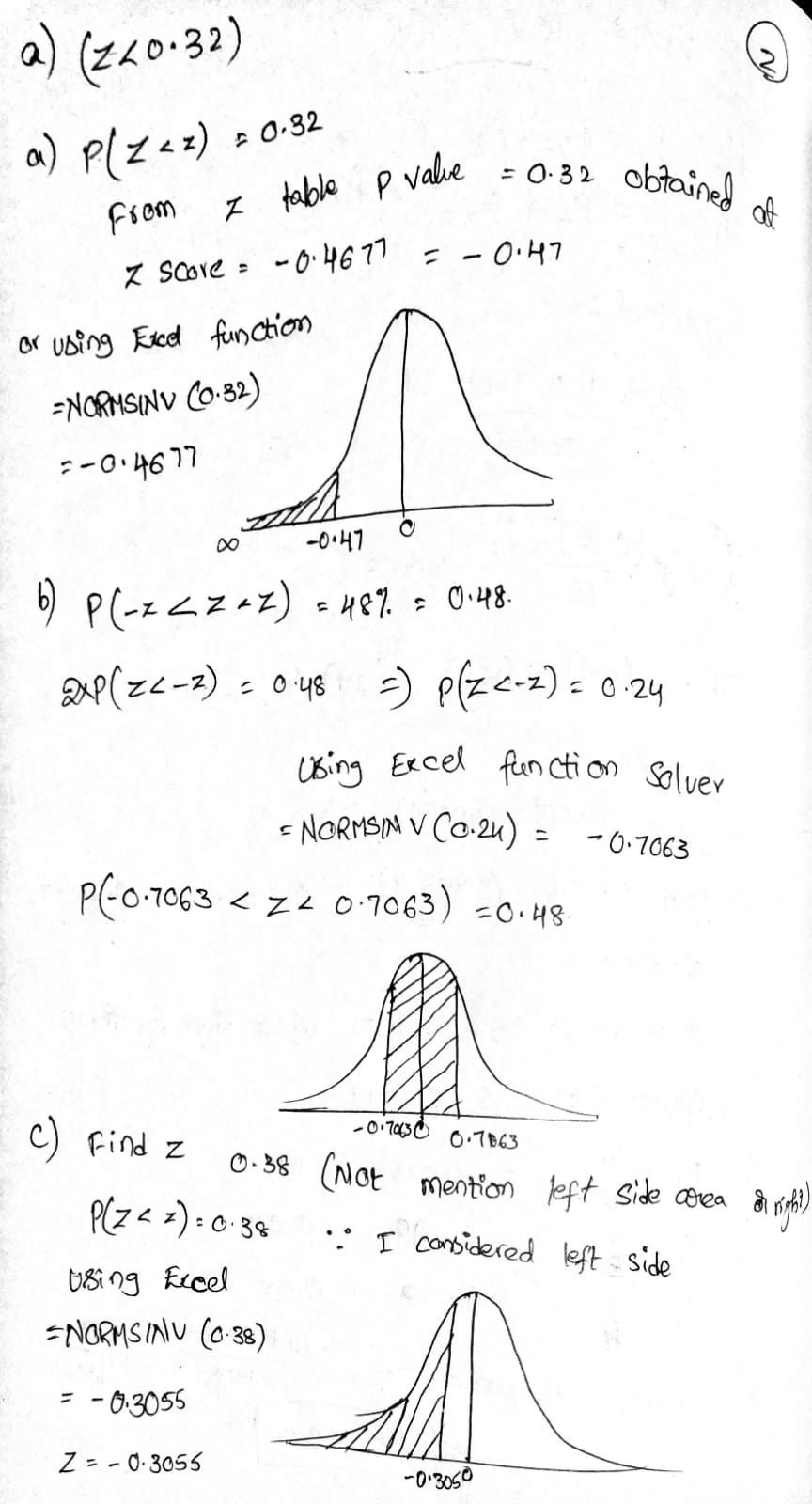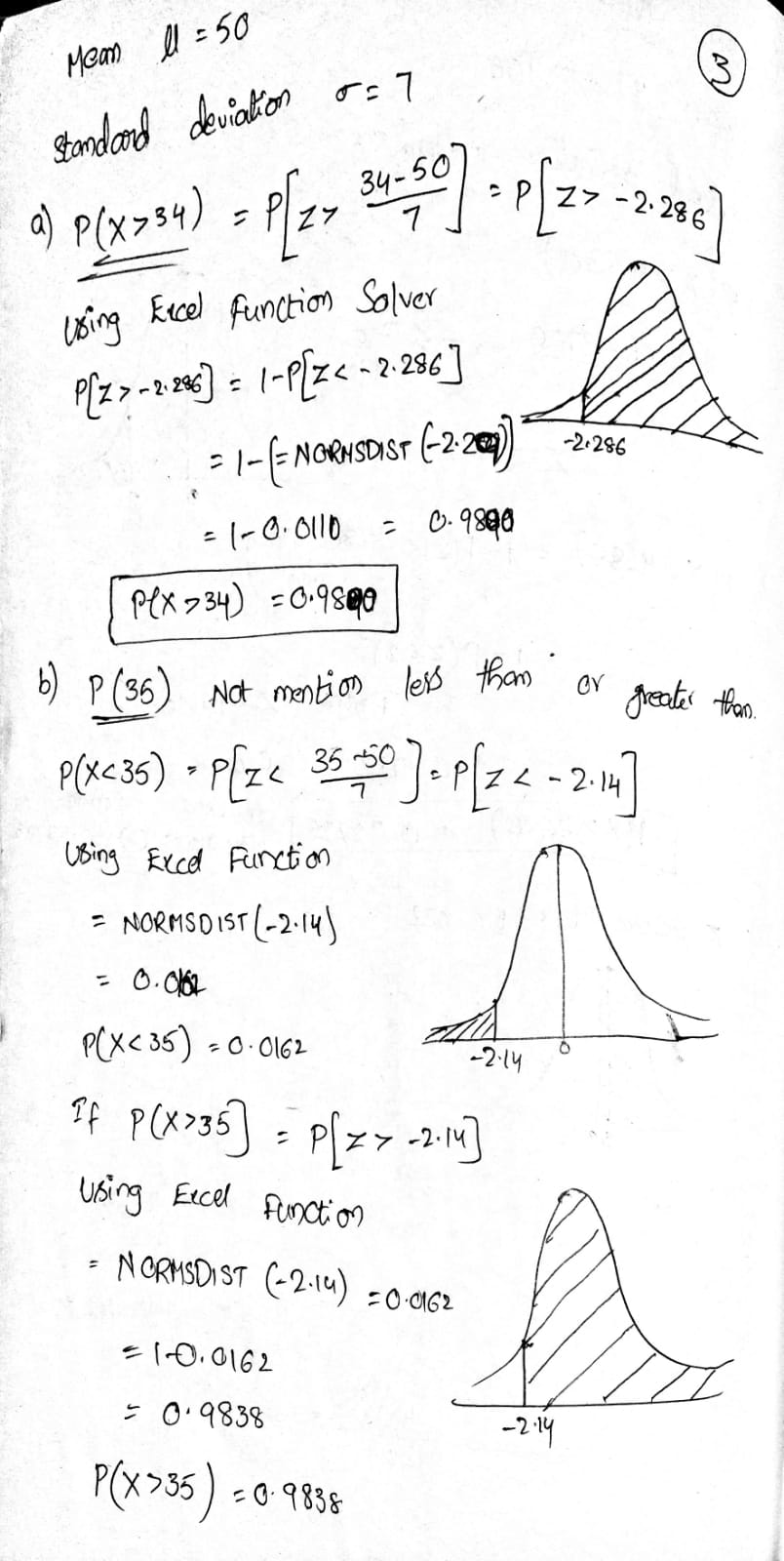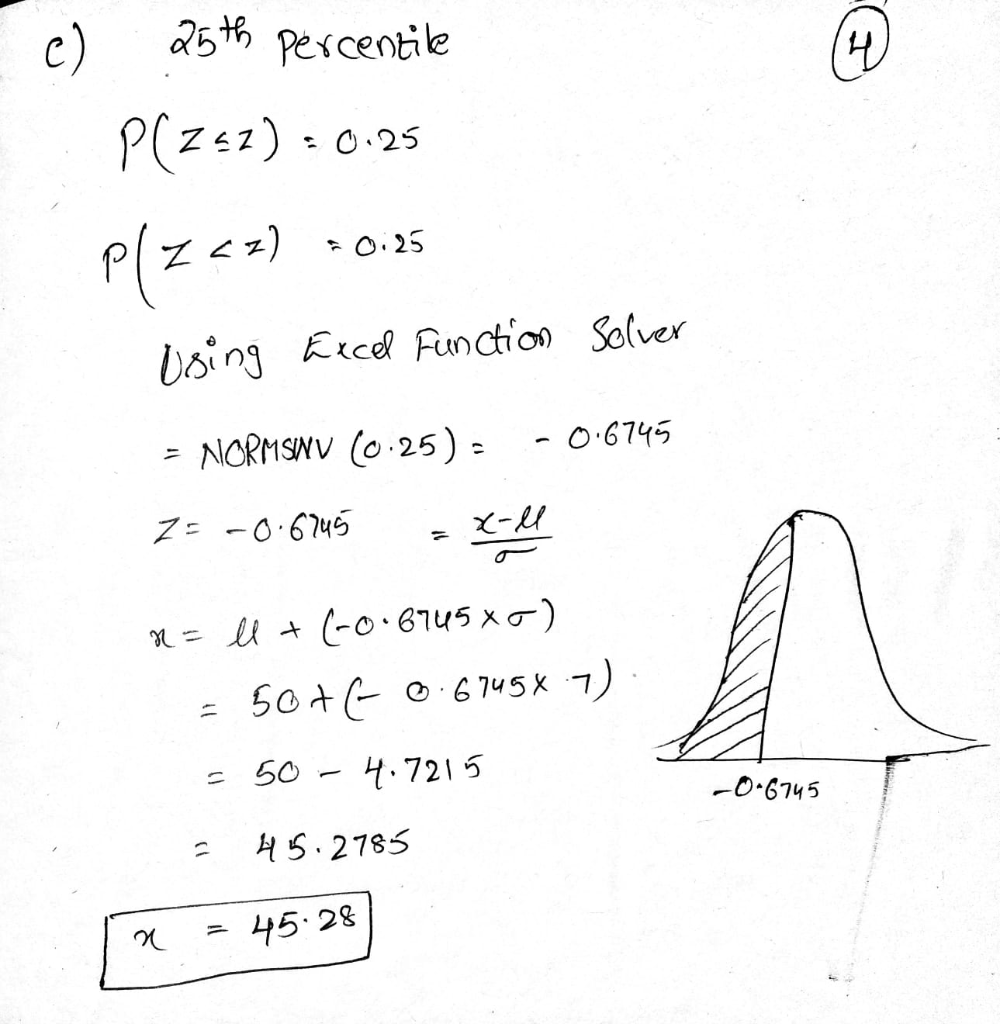#### Earn Coins

Coins can be redeemed for fabulous gifts.

Similar Homework Help Questions
• ### Suppose the birth weights of​ full-term babies are normally distributed with mean 3600 grams and standard...Suppose the birth weights of​ full-term babies are normally distributed with mean 3600 grams and standard deviation σ = 480 grams. Complete parts​ (a) through​ (c) below. Suppose the birth weights of full-term babies are normally distributed with mean 3600 grams and standard de ation σ=480 grams. Complete parts a through c) below. (a) Draw a normal curve with the parameters labeled. Choose the corect graph below O A. C. Ο D. 3120/4080 28404560 264013600 3600 3600 3120 (b) Shade...

• ### 10 of 32 16 complete Suppose the birth weights of full-term babies are normally distributed with...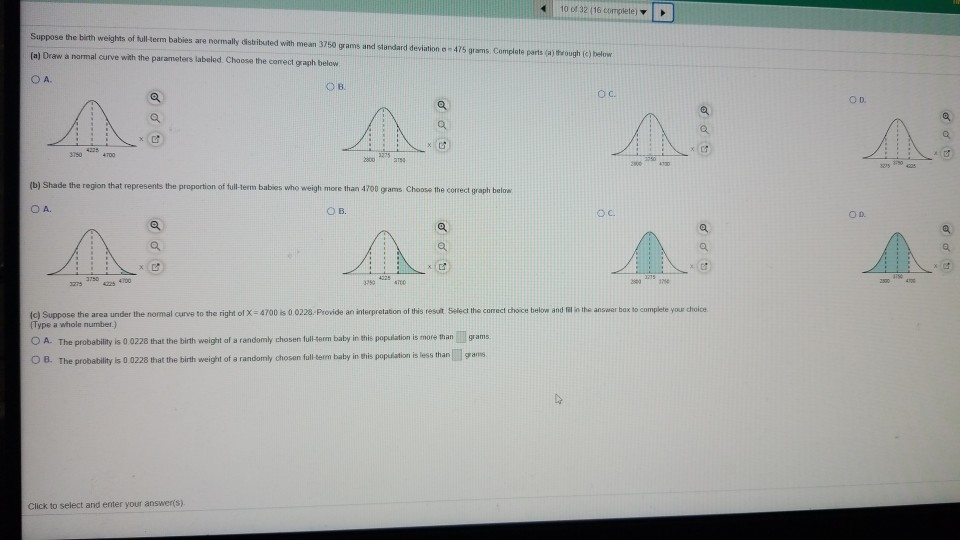10 of 32 16 complete Suppose the birth weights of full-term babies are normally distributed with mean 3750 grams and standard deviation - 475 grams Complete parts (a) through (c) below (a) Draw a normal curve with the parameters labeled. Choose the correct graph below ОА. OB OC OD no a 3150 4335 4700 3975 800 2000 4730 (b) Shade the region that represents the proportion of full-term babies who weigh more than 4700 grams Choose the correct graph below...

• ### birth weights of full-term babies in a certain region are normally distributed with mean 7.125 pounds...

birth weights of full-term babies in a certain region are normally distributed with mean 7.125 pounds and standard deviation 1.290 pounds,find the probability that a randomly selected new born will weigh less than 5.5 pounds

• ### Assume that full-term babies' weights are Normally distributed with a mean of 3500 grams and a...

Assume that full-term babies' weights are Normally distributed with a mean of 3500 grams and a standard deviation of 600 grams. What weights make up the middle 50% of all full-term babies' weights? Round your answers to the nearest gram (the ones place). The low end of the range is grams and the high end is grams.

• ### A particular fruit's weights are normally distributed, with a mean of 745 grams and a standard...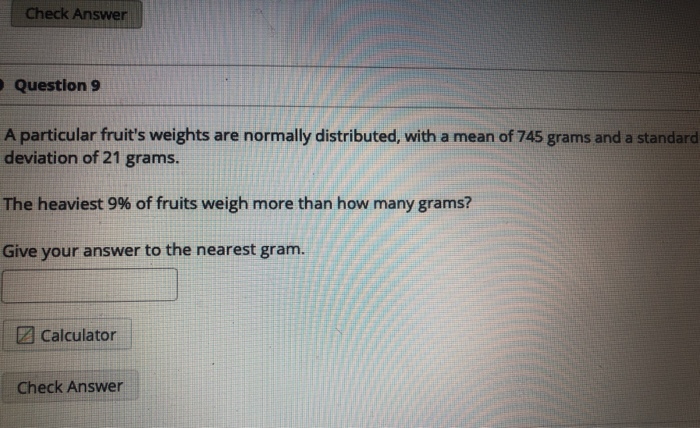A particular fruit's weights are normally distributed, with a mean of 745 grams and a standard deviation of 21 grams. The heaviest 9% of fruits weigh more than how many grams? Give your answer to the nearest gram. Check Answer Question 9 A particular fruit's weights are normally distributed, with a mean of 745 grams and a standard deviation of 21 grams. The heaviest 9% of fruits weigh more than how many grams? Give your answer to the nearest gram....

• ### 1. Suppose we know that the birth weight of babies is normally and standard deviation 500g. (1) W...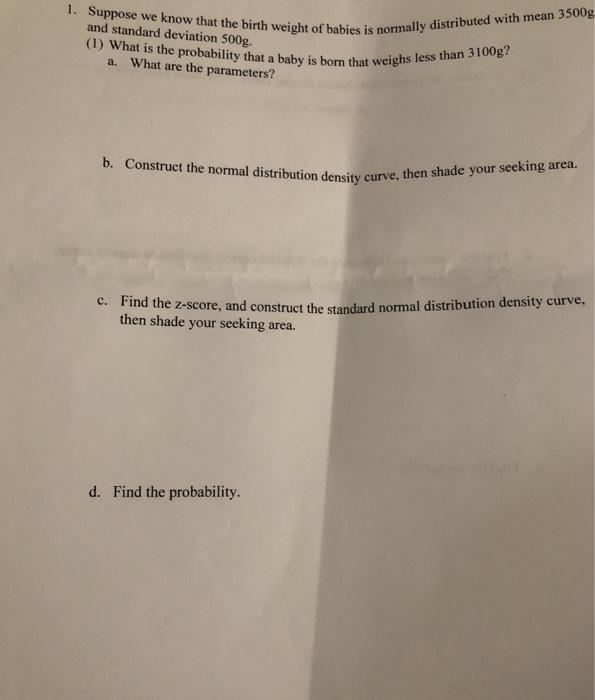1. Suppose we know that the birth weight of babies is normally and standard deviation 500g. (1) What is the probability that a baby is born that wei than 3100g? I t What are the parameters? a. b. Construct the normal distribution density curve. then shade your seeking area. ind the z-score, and then shade your seeking area. construct the standard normal distribution density curve, d. Find the probability. 1. Suppose we know that the birth weight of babies is...

• ### A random sample of the birth weights of 186 babies has a mean of 3103 grams...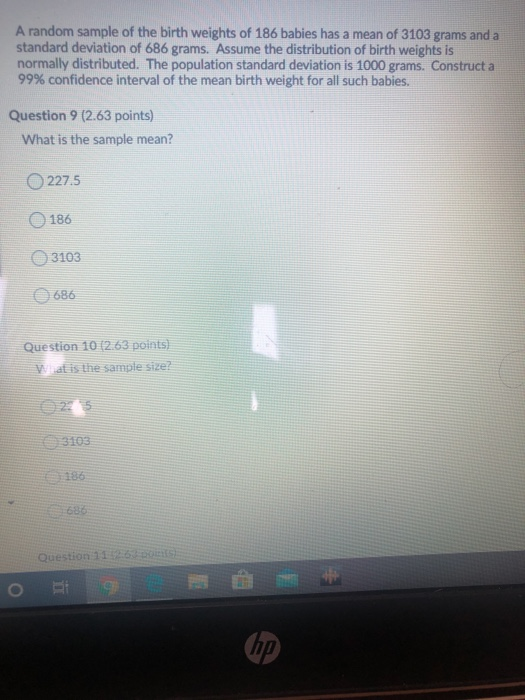A random sample of the birth weights of 186 babies has a mean of 3103 grams and a standard deviation of 686 grams. Assume the distribution of birth weights is normally distributed. The population standard deviation is 1000 grams. Construct a 99% confidence interval of the mean birth weight for all such babies. Question 9 (2.63 points) What is the sample mean? 227.5 186 3103 686 Question 10 (2.63 points) Wat is the sample size? Question 116) BE hp

• ### Birth weights in the United States are normally distributed with a mean of 3420 grams and...

Birth weights in the United States are normally distributed with a mean of 3420 grams and a standard deviation of 495 grams. If we randomly select 36 babies in the U.S., what is the probability that their mean (average) birth weight will be greater than 3500 grams?

• ### Suppose the weights of newborn babies is normally distributed with a mean of 7 lbs and...

Suppose the weights of newborn babies is normally distributed with a mean of 7 lbs and a standard deviation of 1.5 pounds. How much would a baby have to weigh to be in the top 10% of birthweights? a. 8.5 b. 5.08 c. 8.35 d. 7.15 e. 8.92

• ### Assume the random variable X is normally distributed, with mean = 50 and standard deviation 0...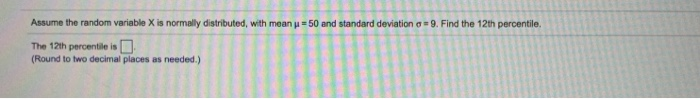Assume the random variable X is normally distributed, with mean = 50 and standard deviation 0 - 9. Find the 12th percentile. The 12th percentile is 0 (Round to two decimal places as needed.) Find the Z-scores that separate the middle 67% of the distribution from the area in the tails of the standard normal distribution Click the loon to view a table of areas under the normal curve. The Z-scores are a (Use a comma to separate answers as...